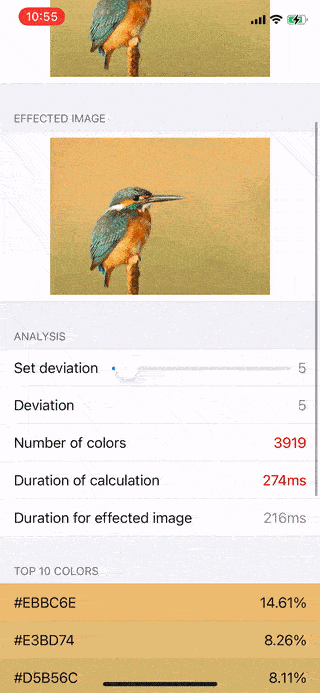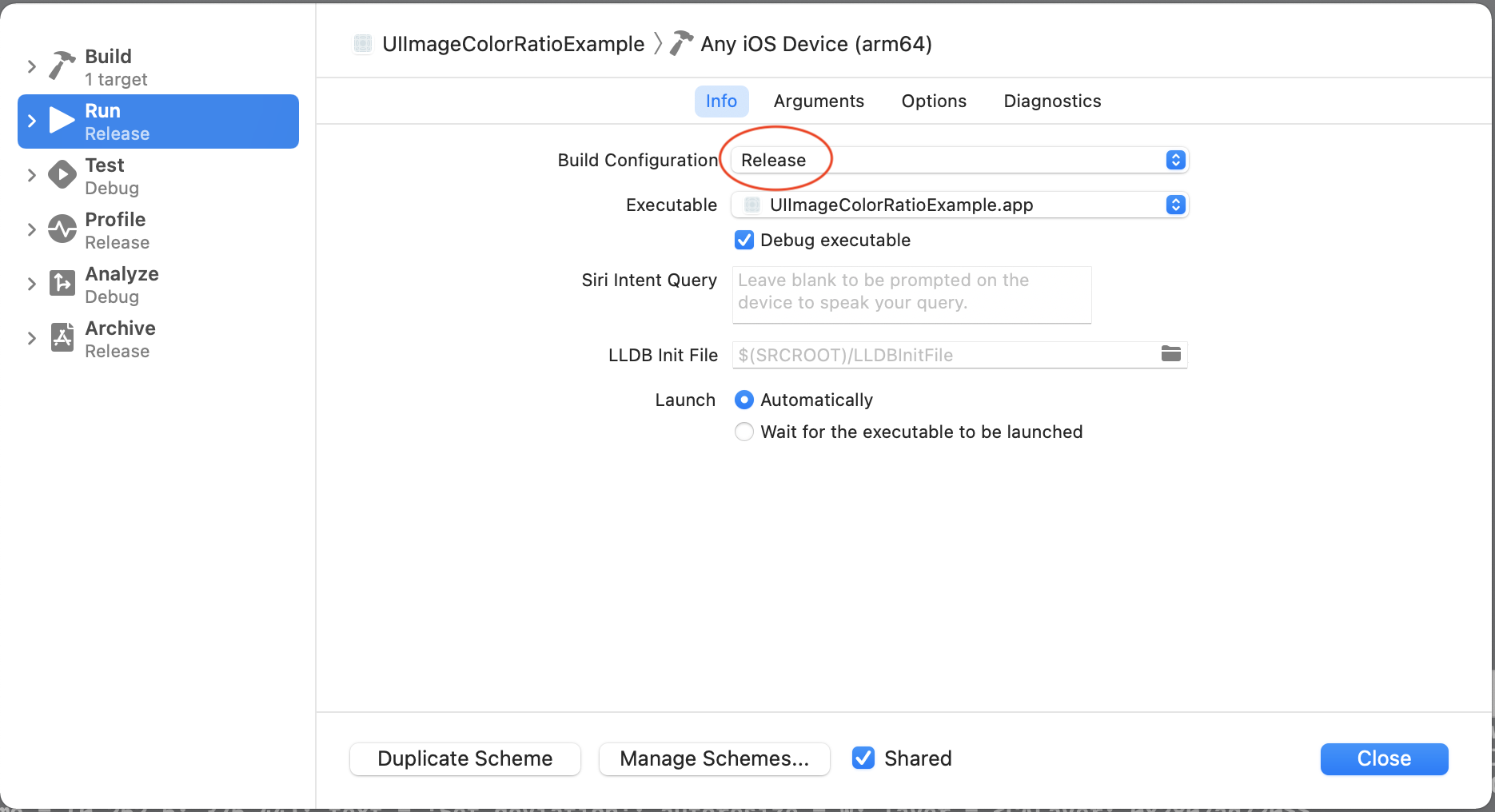# UIImageColorRatio

A tool to calculate the color ratio of UIImage in iOS.# How to use UIImageColorRatio

Get the color ratio of UIImage.

```let image = ...  // your UIImage.
let result = image.calculateColorRatio(deviation: 10) // "deviation": The deviation on pixels, It's from 0 to 255. Bigger deviation means less kind of colors.```

Get the effected image.

``````let image = ...  // your UIImage.
let result = image.calculateColorRatio(deviation: 10)
let effectedImage = image.effectedImage(colorRatioResult: result)
``````

# How to integrate UIImageColorRatio?

UIImageColorRatio can be integrated by cocoapods.

``````pod 'UIImageColorRatio'
``````

Feel free to send Pull Request to support Carthage or Swift Packages.

# Performance

You can see the duration of the calculation from `calculateColorRatio` API’s result.

You MUST use Release build configuration to see the performance. Because swfit is very slow on Debug build configuration.Refer here: https://stackoverflow.com/q/61998649/9315497

# Requirements

• iOS 10.0+
• Xcode 11+
• Swift 5.0+

## GitHub

https://github.com/623637646/UIImageColorRatio